# Formula Fields in Bionic Rules

## Overview

This article reviews the Formula fields supported in Bionic Rules. The Formula fields allow you to create different types of formulas so that you don’t have to create the formula on the Object directly in SFDC or MDA. You can use these Formula fields only when you perform any Transformation task. Formula fields allow you to do the following tasks:

• Build a numerical expression on fields with all arithmetic operators.
• Generate a formula on the columns of a single record.

## Supported Formulas

• Numeric Formulas
• Admin Page Views + Config page views
• Current Score - Previous Score
• NPS Avg * 10
• ((Current Score - Previous Score) / Previous Score) * 100
• String Formulas
• First Name + Last Name
• CTA Comments + Custom Text
• Convert to Upper Case (Account ID)
• LEFT(field, substring) - extracts the left part of the string from the appearance of string character.
• RIGHT(field, substring) - extracts the right part of the string with the appearance of a string character.
• POSITION(field, substring) - provides the position of the substring in a string. For example, POSITION(“doe” in “johndoe”) returns 5. Counting of the position starts with an index 1.
• LENGTH(field) - provides the length of the string. For example, LENGTH(“string”) returns 6.
• SUBSTRING(field, position, length) - finds the substring based on the position and length.
• Date Formulas
• (Last Modified Date - Created Date) in days
• Convert to Date time (Created Date)
• Period Over Period Comparison
• Calculated Fields: For more information on these fields, refer Using Calculated Fields in Rules.
• Case Expression: Allows you to categorize data based on criteria.

### Limitations

• A formula field cannot be added when a field is added to the GroupBy section.
• Constants in Formula Field are not considered as a token.
• Formula time span cannot exceed 2 years.
• Period Over Period Comparison or Period Over Period Comparison in % formula cannot be created unless a date identifier is present.
• Negative values are not allowed in the Function for Period Over Period Comparison and Period Over Period Comparison in %.
• ((A-B) * (A+B)) cannot be simplified to (A-B) * (A+B).
• Period Over Period Comparison and Period Over Period Comparison in %accept only integers, not decimal values.
• Concat function allows only 10 arguments.
• A maximum of 10 formulas can be added.

## Create a Rule for Formula Fields

1. Navigate to Administration > Rules Engine > +RULE.
2. In the Create Rule screen, enter the following:
• Rule For: Company
• Rule Name: Using Formula Fields. This dataset name will be used as the source dataset while creating a Transformation task.
• Folder: Select a folder for the rule.
• (Optional) Description: Provide a description for the rule.1. Click NEXT. The Setup Rule screen appears.
2. Click DATASET.
3. Enter a Task Name: Using fields for formula.
4. Select Usage Data as the Source Object.1. Populate the Show and Filter sections as shown in the image below:1. Click SAVE and navigate to the Setup Rule screen.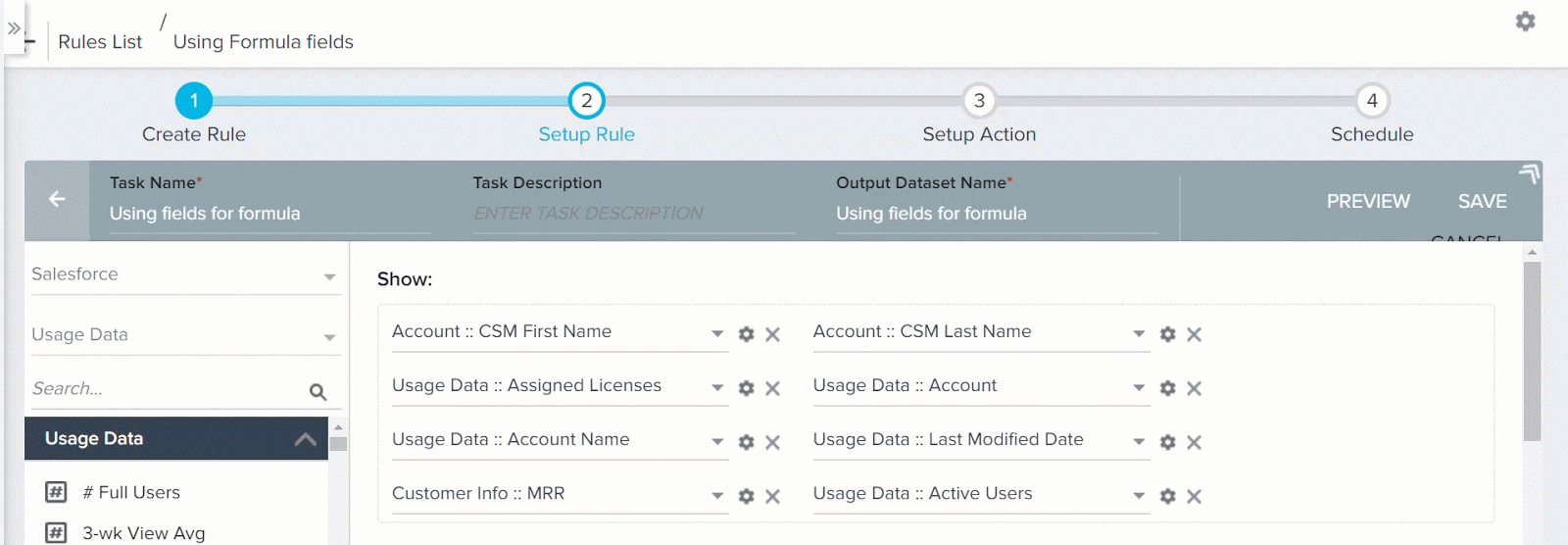It is considered that every Account's data is modified on the rule run date (today). So Last Modified Date field is the same as current date.

### Application of Formulas

1. Click +TASK and select Transformation.

Note: You can use Formula fields only in Transformation Tasks.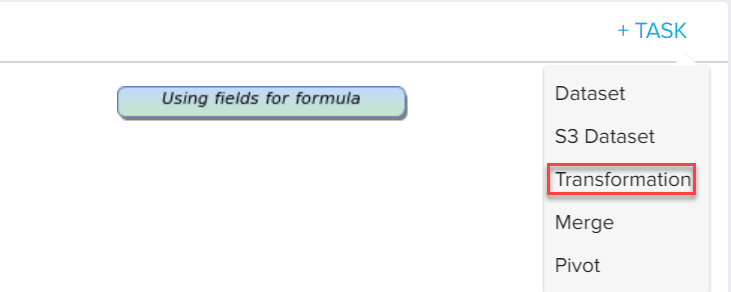1. Enter a Task Name and select the Source Object (here, Using fields for formula).#### String Formulas

The following functions allow only for String, Picklist, Multi-picklist data types. The complete list of String formulas supported by Rules Engine and their description is given below:

1. Concat: Concatenates the selected arguments. A maximum of 10 arguments can be added and one string can be used multiple times.
2. To Lower Case: Converts the selected argument values to Lowercase.
3. To Upper Case: Converts the selected argument values to Uppercase.
4. ​​​​​​LEFT(field, substring): extracts the left part of the string from the appearance of string character. For example, LEFT(johndoe@gainsight.com, @) will return “johndoe” as the output.
5. RIGHT(field, substring): extracts the right part of the string with the appearance of a string character. For example, RIGHT(johndoe@gainsight.com, @) will return “gainsight.com” as the output.
6. POSITION(field, substring): provides the position of the substring in a string. For example, POSITION(“doe” in “johndoe”) returns 5. Counting of the position starts with an index 1.
7. LENGTH(field): provides the length of the string. For example, LENGTH(“string”) returns 6.
8. SUBSTRING(field, position, length): finds the substring based on the position and length. For example, Substring(“johndoe”, 2, 3) returns “ohn”.

You can see the following example on creating String Formula using Concat operation.

The CSM First name and CSM Last Name fields of CSM for every Account is available. Instead of viewing first name and last name separately, you can use String function to concatenate these fields and merge them into a single field called CSM. To perform this in a Transformation task:

1. Expand Formula Fields on the left pane.
2. Expand String Formulas.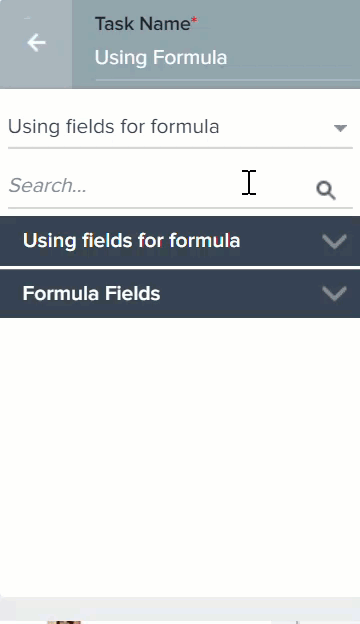1. Drag and drop the formula field to the Show section. Concat function window appears.
2. In the concat function window, provide the following information:
• Output Field Label: Enter only alphanumeric and underscore characters in this field. The label should always start with an alphabetical character.
• Output Header: This name is used as CSV Header to process action. This is view only and is generated based on the Output Field Label (but without space).
1. Perform Concat Operation to create CSM field as shown in the following image.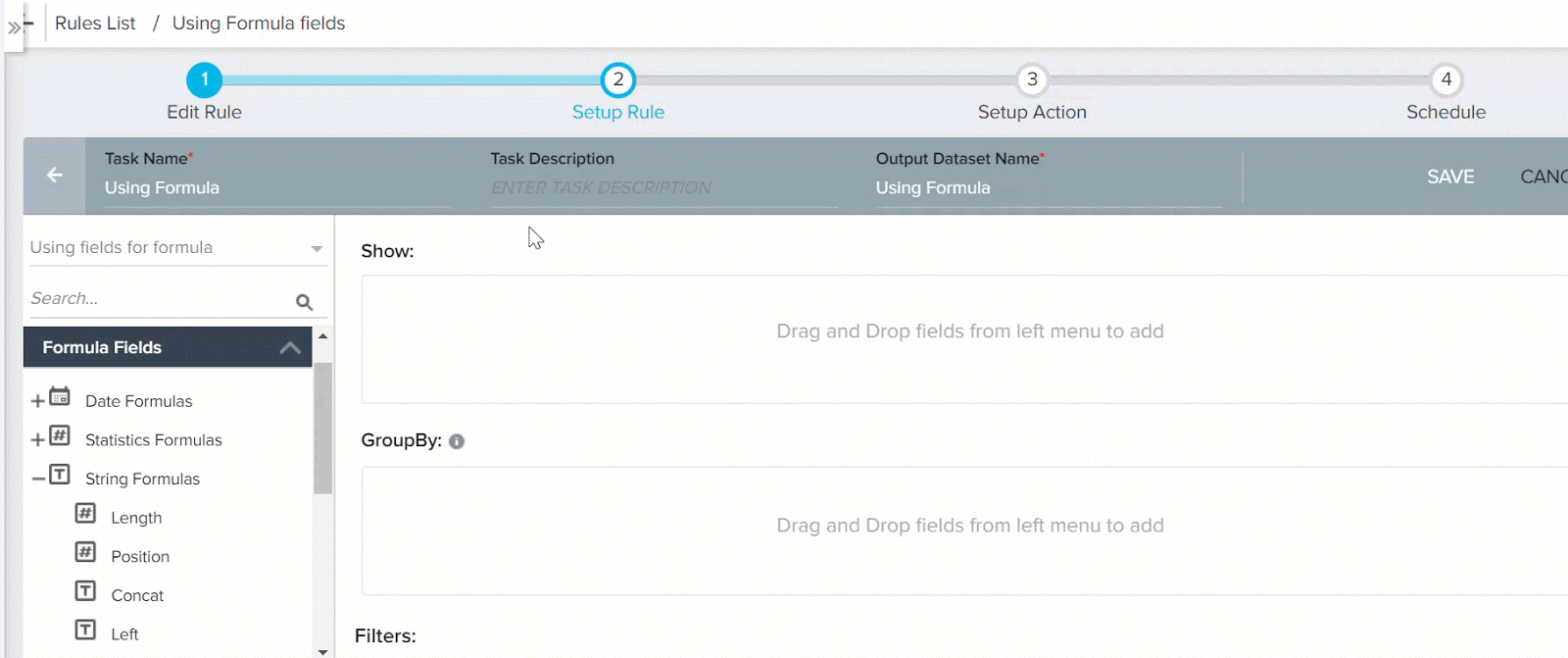In this example, the CSM First Name and CSM Last Name fields are concatenated and a new column CSM is created. The CSM column stores the full name of the CSM.

Formula used: CSM = CSM First Name + CSM Last Name

1. Click SAVE. Clicking RESET erases the data that you entered and allows you to refill the field with new information.
2. In the Setup Rule page, you can see the newly created Formula Field in the Show section as shown in the image.

#### Date Formulas

The complete list of Date formulas supported by Rules Engine and their description is given below:

The following functions allow only for Datetime data type.

• Date: Converts Date-time argument to Date.
• Date Diff: This function returns the the difference between two selected dates. The output is always returned in numerical format (number of days).

The following functions allow only for Date and Datetime data types:

• First Day of Calendar Quarter: Returns the first day of Calendar Quarter.
• First Day of Current Month: Returns the first day of Current Month.
• First Day of Current Week: Returns the first day of Current Week.
• Last Day of Calendar Quarter: Returns the last day of Calendar Quarter.
• Last Day of Current Month: Returns the last day of Current Month.
• Last Day of Current Week: Returns the last day of Current Week.
• Add/Subtract Date: You can use this function to perform addition and subtraction operations on a date. These operations can be performed either on a date used in the dataset, or on the rule execution date. You can add or subtract any number of days or weeks to a date.

To use this function:
1. Expand Date formulas.
2. Drag and drop Add / Subtract Date to Show section.
3. Enter a name in the Output Field Label field
4. Select a date field either form the Dataset or Rule Date.
5. Select either the + or - operator.
6. Enter a number in the Value field.
7. Select either Days or Weeks in the Value field.
8. Click SAVE.• Rule Date: The Rule Date function does not accept any arguments. It only returns the Current Date on which the rule is being executed. You can also use the Rule Date function as an argument in any of the above functions. However, for the Date Diff function, you can use Rule Date as an argument only in the end datetime field, and not in the start datetime.You can see the following example on creating Date Formula.

You can use Date formula to calculate number of days left for license renewal. To perform this in a Transformation task:

1. Expand Formula Fields on the left pane.
2. Expand Date Formulas.
3. Drag and drop Date Diff to Show section. Date Diff formula field window appears.

Note: Date Diff function returns the difference (in number of days) between start datetime and end datetime fields.

1. In the Date Diff formula field window, provide the following information:
• Output Field Label: Enter only alphanumeric and underscore characters in this field. The label should always start with an alphabetical character.
• Output Header: This name is used as CSV Header to process action. This is view only and is generated based on the Output Field Label (but without space).
• Decimal Places: Enter a number between 0 and 9.
• Get Signed Value: This option is applicable only for Date Diff function. If you select this option, the output of Date Diff function can be a negative integer. However, if you do not select this option, and if Date Diff function has a negative output, it is automatically converted to a positive integer.
For example, if your start datetime value is 5 Jan 2018 and end datetime value is 2 Jan 2018, Date Diff function subtracts start datetime from end datetime. So in this case, the output would be -3 days. If you do not select the Get signed value option, the output is displayed as 3 days. However, when you select the Get signed value option, the output is returned as -3.
1. Perform Date Diff operation as shown in the following image.In this example, a new column Days left until renewal is created. This column contains the difference value (in number of days) after subtracting the Renewal Date from Rule Date (assumed to be current date). If any negative values are displayed in the output, it implies that the renewal date for that customer has been missed.

• Renewal Date: The Date on which the Customer license is scheduled to renew.
• Rule Date: The date on which the rule is being executed. Generally, this reflects today's date. However, if you are running the rule historically, this field will not display today's date.
1. Click SAVE. Clicking RESET erases the data that you entered and allows you to refill the field with new information.
2. In the Setup Rule page, you can see the newly created Formula Field in the Show section as shown in the image.

#### Using Numeric Expressions

You can use the numeric expression to perform various arithmetic calculations on data. You can see the Numeric Expressions on the left pane in the Setup Rule page. The following example on Numeric expressions guides you in calculating the number of used licenses for a customer. The number of used licenses is the difference between the Total number of assigned licenses and the number of Available Licenses. Following is the formula to create a Numerical Expression:

Number of Licenses used = Assigned Licenses - Available Licenses

To create Numerical Expression for this formula:

1. Drag and drop the Numeric Expression to the Show section. The Numeric Expression window appears.
2. In the Numeric Expression window, enter the following:
• Output Field Label: Enter only alphanumeric and underscore characters in this field. The label should always start with an alphabetical character.
• Output Header: This name is used as CSV Header to process action. This is view only and is generated based on the Output Field Label (but without space).
• Decimal Places: Enter a number between 0 and 9.
• Treat null as zero: Select this to replace any null field values with zero while performing calculations using the expression provided. Deselect this to use any null value as is in the calculations.
• Select:
• Assigned Licenses field
• - (subtraction) Operator
• Available Licenses field
1. Perform Numerical Expression as shown in the following image.In this example, a new column Number of Licenses used, is created. This column contains the difference value between the Assigned licenses and Available Licenses. For instance, if Assigned Licenses for a Customer is 10 and Available Licenses is 6, it shows the customer has purchased 10 licenses. However, 6 of the 10 licenses are yet to be used and only 4 licenses are currently operational.

• Assigned Licenses: The number of licenses purchased by the customer.
• Available Licenses: The number of licenses which are not currently used by the customer, out of the total licenses purchased.
1. Click SAVE. Clicking RESET erases the data that you entered and allows you to refill the field with new information.
2. In the Setup Rule page, you can see the newly created Formula Field in the Show section as shown in the image.

#### Period Over Period Comparison Functions

The following are the other available functions:

• Period Over Period Comparison
• Period Over Period Comparison in %

These functions cannot be created unless a suitable field is included in the Group By section. However, if you use any field in the Group By section, existing formula fields that were used so far show up as an error message. So it is recommended that you create another transformation task to use Period over Period comparison functions.

You can use the Period over Period Comparison to create a field which stores average MRR value in percentage over a period of time against each Account.

To use Period over Period Comparison in % to calculate average MRR:

1. Click + TASK and select Transformation.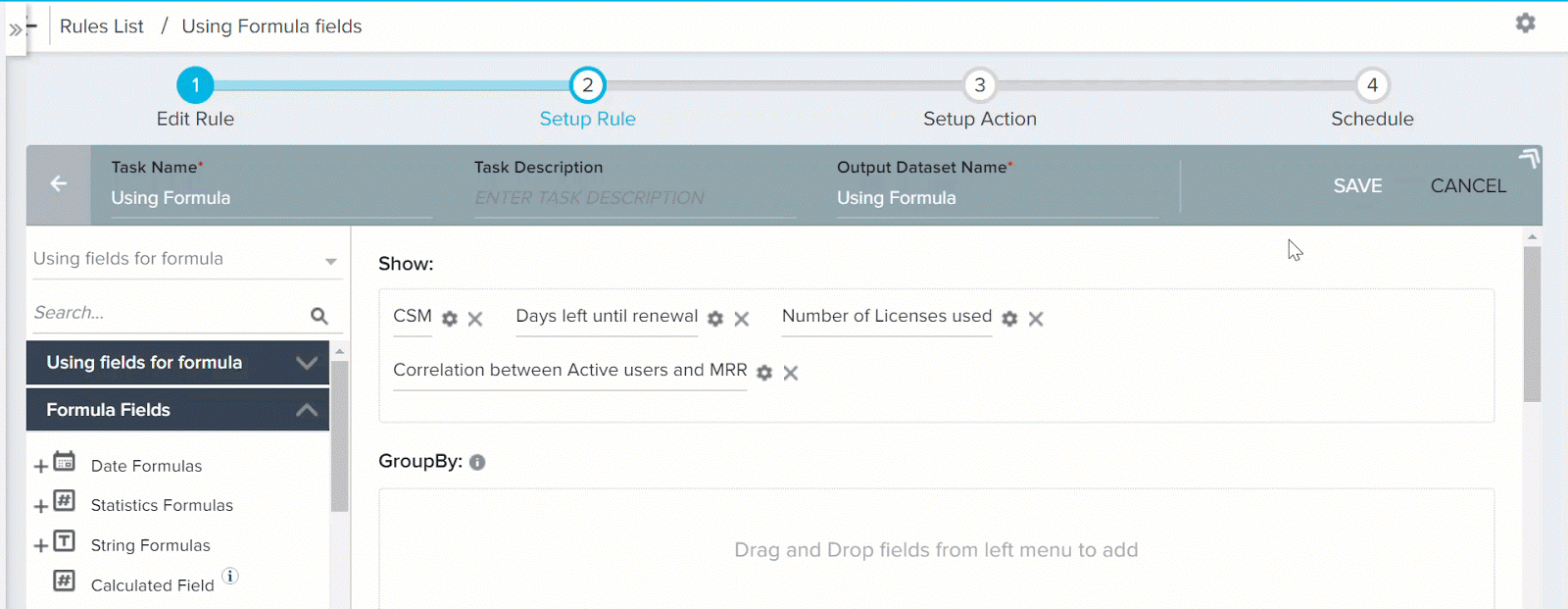1. Enter a name in the Task Name field.
2. Select the Source task. (here Using fields for formula)
3. Drag and drop Account Name field to the GroupBy section.
4. Drag Period Over period Comparison In % to the Show section. Period Over period Comparison In % configuration dialog appers.
5. In the Period Over period Comparison In % configuration dialog, enter the following:
• Output Field Label: Enter only alphanumeric and underscore characters in this field. The label should always start with an alphabetical character.
• Output Header: This name is used as CSV Header to process action. This is view only and is generated based on the Output Field Label (but without space).
• Decimal Places: Enter a number between 0 and 9.
1. Perform Period Over period Comparison In % configuration as shown in the following image.1. Enter the following values in the Period Over period Comparison In % configuration:
• Period Type: Months
• Duration: 12
• Metric: MRR
• Aggregation Method: AVG
• Num of Prior Periods: 1
• Ignore Missing Data: True
1. Click SAVE. Clicking RESET erases the data that you entered and allows you to refill the field with new information.
2. In the Setup Rule page, you can see the newly created Formula Field in the Show section as shown in the image.This function calculates average MRR for the past year (in percentage) for all the Accounts. The output table consists of Avg MRR for past 12 months (in percentage) grouped against every Account.

The Period over Period Comparison function also performs similar task like Period Over period comparison in %, but it considers actual numerical values and not percentage values.

Example: Function syntax is as listed below. In this case, this function has a fixed number of arguments and each argument has its own context.

• Period Over Period Comparison ( Period([ DAY / WEEK / MONTH / YEAR ], <Duration>),
• <Metric [ field of type numeric ]>,
• <Date Identifier [ field of type date ]>,
• <Aggregation Method [ SUM,AVG,COUNT, COUNT DISTINCT, MIN, MAX ]>,
• <Num of Prior Periods>,< Treat Missing Data as Zero  [ true/false ]>);

For more information on the Period Over Period Comparison functionality, refer Bionic Rules Period Over Period Comparison.

#### Statistical Functions

Gainsight introduces Statistical Functions in Rules Engine. This is a first step towards introducing Data Science capabilities in the Rules Engine. Using Statistical Functions, you can derive insights from your datasets that will help you make more informed decisions regarding your product and customers.

The complete list of Statistical functions supported by Rules Engine are:

1. Correlation - The correlation function shows you how two variables are related. This function looks at the strength of the relationship between two variables and helps you make a decision based on the results.
2. Covariance - Covariance is a type of correlation. It indicates whether there is a positive or negative correlation but does not indicate the strength of correlation between two variables.
3. Standard Deviation and Variance - Both Standard deviation and variance measure volatility/variability. A high value indicates that the dataset is spread out; a low standard deviation means that the dataset values are close to the mean.
4. Variance - Variance measures how far a set of numbers are spread out from their average value.
5. Median - Median is more useful than an average when your dataset has outliers; meaning if the dataset has high standard deviation.
##### Best Practices in using Statistical Functions
• Correlation and Median functions, if used together within the same task, may not provide accurate results if the data has nulls.
• Add filters with not null checks: if you are unsure if the dataset has null values or not and want to use correlation along with other statistical functions within the same task, it is suggested to add filters with not null checks. This will filter out the null data records and produce accurate results.
• Correlation/Median with other aggregations: It is suggested not to use correlation or median functions in conjunction with any other aggregation (like sum, min, max, avg) or function if your data has null values.
• For Nulls: Use "Treat null as Zero" for all the relevant metrics. You can use formula field here to pick each of these fields and select the Treat null as Zero option.
##### Correlation

The correlation function shows you how two variables are related. This function looks at the strength of relationship between two variables and helps you make a decision based on the results. For example, this function lets you forecast a risk and helps you take appropriate steps.

For example, if you see the data of two variables and correlate it, if one variable goes up and the other goes down, then it is said to have a negative correlation. In this case, you can be assured that risk is not involved based on the two variables in consideration. If one variable goes up and the other goes up, then it is said to have a positive correlation. In this case, you can decide that the two variables have a correlation and then take the necessary steps to mitigate the risk.

For example in Gainsight, you can correlate the number of CTAs in a high-risk category (1st variable) and the customer churn in the next 4 months (2nd variable). If the result says that they have a positive correlation, then you can take appropriate steps to understand why the customer is churning.

Following are some use cases in which Statistical Functions can be used:

1. Are the number of support tickets positively/negatively correlated to a higher NPS score?
2. Is health score correlated to Churn Rate or Upsell Opportunity?
3. Are the Number of Active Users correlated to the MRR obtained from that customer?

Prerequisite: A field with Number datatype should be present to use this function.

Output: any value between -1 and +1. Can also be a decimal.

In this example, the third use case is depicted. You can use Correlation function to test the relation between Active Users and MRR (Monthly Recurring Revenue). Correlation showcases if there is any relation between two variables. Based on the results obtained, you can infer if there's a relationship between the MRR obtained from a customer and the number of Active Users.

To calculate correlation between Active Users and MRR in a Transformation task:

1. Drag and drop Correlation to the Show section. Correlation function configuration window appears.
2. In the Correlation function configuration window, provide the following information:
• Output Field Label: Enter only alphanumeric and underscore characters in this field. The label should always start with an alphabetical character.
• Output Header: This name is used as CSV Header to process action. This is view only and is generated based on the Output Field Label (but without space).
• Decimal Places: Enter a number between 0 and 9.
1. Select Active Users and MRR fields in the Correlation configuration as shown in the image below:1. Click SAVE. Clicking RESET erases the data that you entered and allows you to refill the field with new information.
2. In the Setup Rule page, you can see the newly created Correlation Field in the Show section as shown in the image.
3. To view the results of the functions, save the task and run the rule. In the execution history, you can see the task’s output and download them.

A new column Correlation between Active users and MRR is created. This column contains a value in the range between -1 and 1.

In real world scenario, the number of Active Users may be directly proportional to MRR. Thus, an increase in Active Users may result in an increased MRR from the customer. Hence, it is expected that there exists a positive Correlation between the Active users and MRR fields.

When you use two transformation tasks, it is essential to use a Merge task to combine the two transformation tasks and create a output dataset having output fields from each transformation task, before performing an Action.

#### Case Expression

You can use the Case Expression formula field to categorize data, based on specific set of requirements. You can use this Formula function to create an output Field. This output field has values for the records that match your specific set of requirements.

Anatomy of Case Expression:

• Case Expression function is made up of multiple cases (a maximum of 10).
• Every Case consists of multiple Criteria (a maximum of 5). Each criteria is a specific requirement that any record should match. For example, a Criteria for a customer to be classified as Detractor can be NPS score between 0-6.

Every Case has an associated Action when any record matches with the given criteria. A value (Ex: Detractor) is populated in the Output field. This value can be a custom value or fetched from another field in the source dataset.

Execution of the Case Expression in detail:

1. Execution of the Case Expression begins with the evaluation of the first case on a record. If all the criteria in this case are satisfied by the record, the action associated with this case is executed. The execution of Case expression halts here for this record and none of the other cases are evaluated.
2. However, if the first case is not satisfied, the system evaluates the second case on the same record, and so on. If none of the available cases are satisfied by the record, the default case is executed.

This process is applied to all the records.

The Default Case: The Case Expression also has a default case. This default case does not have any criteria. It only has an action; Default action. You cannot delete the default case. When a record does not match any of the specified criteria, the action associated with the default case is executed.

The various fields present in Case Expression formula field are:

• Output Field Label: Enter the name for the Output column. This name is displayed on the final output page for the Case Expression result.
• Output Header: This is a view only field. The name is derived from the name given in the above field.
• Output Data Type: Select the data type for the output. The options present in the Case Output depends on the Output data type. Three data types are available:
• Number
• String
• Boolean
• Decimal Places: If you select Number in the Output Data Type, select the number of decimal places upto which the output must be displayed, for floating point numbers.
• Case: Case consists of the Criteria(s). You can add multiple criteria by clicking +
• Criteria. The various fields present in a Criteria are:
• Field: Select the field on which the Criteria must be applied. All the fields included in the Dataset are displayed here.
• Operator: Select an Operator to be applied on the field. The operators are displayed based on the field selected.
• Matching Criteria: Select the matching criteria which must be satisfied, for this criteria to be successful. You can either select:
• Value: Enter a value which must match the field value.
• Field: Select a field whose value must match the value of the field, selected in the first field.
• Null records check box: Select this check box to include records which are Null.
• Advanced Logic: When you have multiple criteria, select the logical operator to be applied between those criteria(s). By Default, the AND logic is applied.
• Then: If all the criteria(s) are matched, the action specified in this field is executed. The options available in this field depend on the value selected in the Output Data Type field.
• Default: This field represents the default case. There is no criteria for this case. It only has action. If all the cases fail, this case is executed.

In the below example, an output field called Customer on Verge of Churn is created. The output type for this is Boolean. So if there is a customer whose MRR value less than 1000 AND Trend Indicator field shows Down, this field displays true value for such customers. For rest of the customers, it displays false , which is the Default case Action.The source input for this test scenario is as shown belowIn this example only 12 customers are included and two evaluation fields are chosen; MRR and Trend indicator. However, in real world with multiple customers and more number of evaluation fields, it becomes cumbersome to identify which customers are on the verge of getting churned. This task is easily accomplished by using Gainsight’s Case Expression formula field.

Case Working method: Case Expression evaluates all the 12 customers to check who matches the case. The first customer Abb Corp Ltd. is evaluated to check if the customer satisfies this case. Since ABB corp does not match any of the two given criterias (MRR not less than 1000 and Trend not equal to Down), this customer does not satisfy Case 1. If there were multiple cases, Case Expression would have had evaluated Abb Corp Ltd. to check if it satisfies some other case. Since there are no further cases to be evaluated, the default case is executed for Abb Corp Ltd. and false value is assigned in the Output column for this customer. This process is repeated for all the 12 customers.

Note: You can include Customer Name field also in the Show section of Transformation task to easily map customer and his Churn status. In the output image below, you can see that five customers are on the verge of getting churned.For a detailed step by step procedure on usage of Case expression, refer this tutorial.